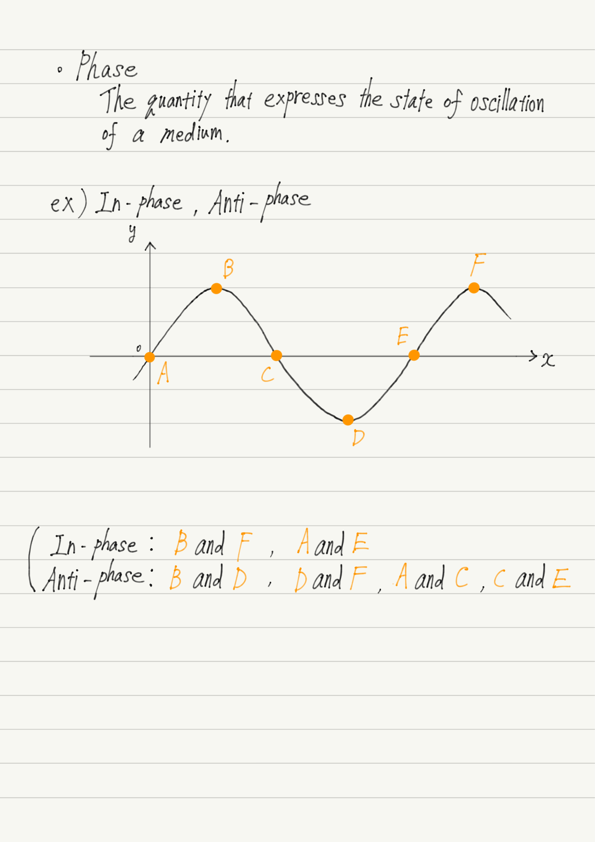# In-Phase and Anti-Phase

In this lecture, “phase”, one of the wave terms whose meaning is particularly difficult to understand, will be briefly explained.

## What is phase?

The textbook says, “The quantity that expresses the state of oscillation of a medium is called the phase.”
But it’s hard to understand, isn’t it?

Therefore, I would like to give a concrete example here.
The concrete examples are “In-phase” and “Anti-phase”.

Phase is used to compare the vibrational state of two different points in a medium.
The most obvious of these cases is when the phases of two points are the same(In-phase) or opposite(Anti-phase).

When you first learn about waves, this is all you need to know for now!
It would be faster to have you look at the summary note than to explain this in words, so I will summarize it here.

## Summary of this lectureAs shown in the summary note, B and F in the figure are in-phase.
These are both wave crests.
This is what is meant by “in the same state of oscillation”.

In the same way, the troughs are in-phase with each other.

On the other hand, for B and D (or D and F), the crest and trough are opposite, so they are in anti-phase.

However, do not make the mistake of thinking that the distinction is based on the value of the y-coordinate.
Given the phases outside of the crest and trough, it turns out to be wrong.

For example, what is the relationship between A, C, and E, the intersections with the x-axis?

The displacements of these points are all the same at y=0.
But, the phases are not all the same.

The same intersection with the x-axis is distinguished by whether it intersects when the graph is up-right (A, E) or down-right (C).

Thus, A and E are in-phase, A and C (or C and E) are in anti-phase.

This time we focused on in-phase and anti-phase.
The exact definition of phase will be explained again when the study progresses.

## Next Time

Learn about the classification of waves.

https://www.yukimura-physics.com/entry/wave-f08

error: Content is protected !!